# Test: Fractional KW Motors of Electrical Machines- 1

## 10 Questions MCQ Test Topicwise Question Bank for Electrical Engineering | Test: Fractional KW Motors of Electrical Machines- 1

Description
Attempt Test: Fractional KW Motors of Electrical Machines- 1 | 10 questions in 30 minutes | Mock test for Electrical Engineering (EE) preparation | Free important questions MCQ to study Topicwise Question Bank for Electrical Engineering for Electrical Engineering (EE) Exam | Download free PDF with solutions
QUESTION: 1

### A 125 W, 4-pole, 110 V, 50 Hz single phase induction motor delivers rated output at a slip of 6%. The total copper loss at full load is 25 Watts. Rotational loss may be assumed to be 25 Watts. Neglecting stator copper loss, the full-load efficiency of the motor would be:

Solution: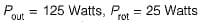Total copper loss; Pcu = 25 Watt
∴ Input power to motor,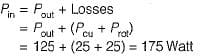∴ Efficiency of motor,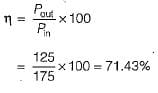QUESTION: 2

### The stepping angle for a 3 - stack, 16-tooth variable reluctance motor is:

Solution:

Number of stacks or phases, n =3
Number of teeth or poles, P= 16
∴ Stepping angle,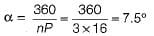QUESTION: 3

### Consider the following statements related to an universal motor: 1. An universal motor runs on d.c. as well as on a.c. 2. No extra winding is required in a universal motor for ac operation. 3. The direction of rotation of universal motor can be reversed by reversing either the field or armature leads, but not both. 4. Universal motors are designed for operation at low speeds (less than 3500 rpm). Of these, the correct statements are:

Solution:

⇒ The compensating winding is required in an universal motor for ac operation to neutralize reactance voltage.
⇒ Universal motors are designed for operation at high speeds (> 3500 rpm).

QUESTION: 4

An 8-pole, 50 Hz, single phase induction motor is running at 690 rpm. It’s slip w.r.t. backward and forward fields respectively are:

Solution: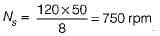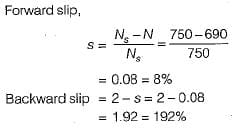QUESTION: 5

Single phase ac motors are classified on the basis of

Solution:
QUESTION: 6

In an induction motor

Solution:
QUESTION: 7

The torqued eveloped by a single phase induction motor drops to zero at

Solution: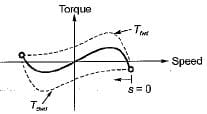From above T-ω characteristic, T= 0 at speed = 0 and slightly below Ns.

QUESTION: 8

In a single phase induction motor

Solution:
QUESTION: 9

In a comparative study of the torque-slip characteristic of a balanced poly-phase induction motor and that of a single phase induction motor, it is found that for zero slip

Solution: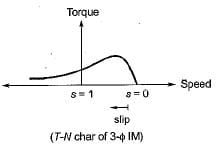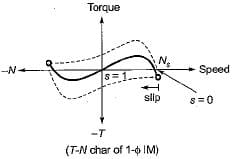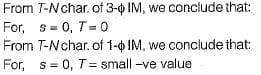QUESTION: 10

Double revolving field theory is based on the idea that pulsating field produced in single phase motors can be resolved into two components of ______ its amplitude and rotating in______ direction with synchronous sped .

Solution:Use Code STAYHOME200 and get INR 200 additional OFF Use Coupon Code